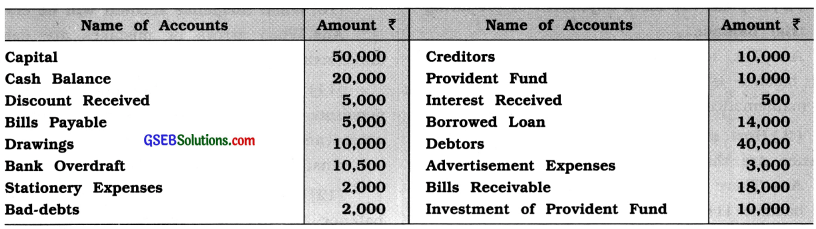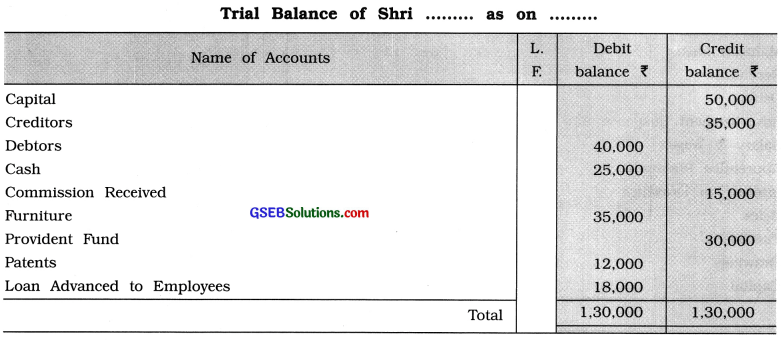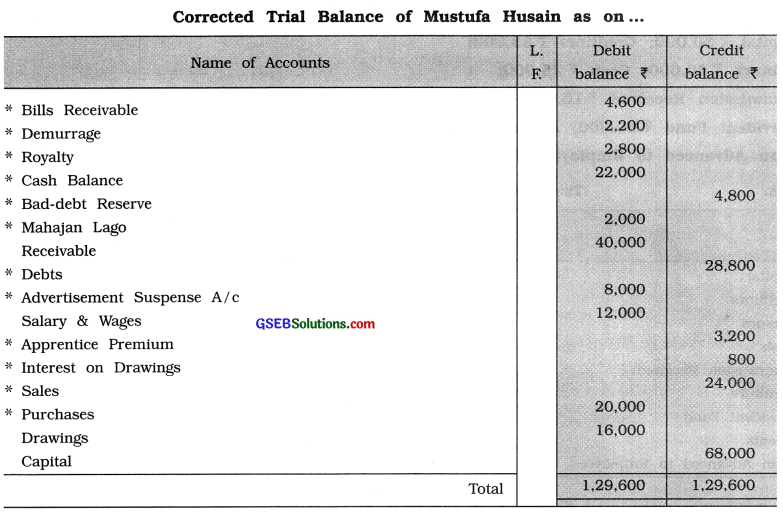# GSEB Class 11 Accounts Important Questions Part 1 Chapter 10 Trial Balance

Gujarat Board GSEB Class 11 Commerce Accounts Important Questions Part 1 Chapter 10 Trial Balance Important Questions and Answers.

## GSEB Class 11 Accounts Important Questions Part 1 Chapter 10 Trial Balance

Very Short Questions (VSQs)
Answer the following questions in one sentence : [1 mark each]

Question 1.
Explain the meaning of trial balance.
A trial balance is a statement prepared at the end of a definite accounting period, showing the totals of the debit and credit sides of each account of the business or their balances.

Question 2.
Explain the main objectives of preparing the trial balance.
The main objectives of preparing the trial balance is to check whether the accounts are mathematically correct or not.Question 3.
How many methods of preparing trial balance are there? Which are they?
There are two methods of preparing trial balance :

1. Totalling method and
2. Balancing method.

Question 4.
Explain any two characteristics of trial balance.
Characteristics of trial balance :

1. The trial balance is a statement, not an account.
2. The trial balance is prepared on a definite date at the end of a definite accounting period.

Question 5.
Why is trial balance prepared ?
Trial balance is prepared to find out mathematical accuracy of the business transactions recorded in the books of accounts and to facilitate preparation of the final accounts.

Question 6.
On which date is a trial balance prepared ?
Generally, a trial balance is prepared at the end of accounting year.

Question 7.
What are the forms of trial balance ?
There are two forms of trial balance viz.,

1. Statement form and
2. Account form.

Question 8.
On the which basis, trial balance is prepared ?
Trial balance is prepared on the basis of all the accounts of ledger.Question 9.
When trial balance is prepared ?
Trial balance is prepared before the preparation of the final accounts.

Question 10.
When Suspense account will be closed?
When errors or mistakes are detected and rectified, Suspense account will be closed.

Question 11.
“If the trial balance agrees, the accounts are absolutely correct.” Explain the correctness of this statement.
This statement is False.

Question 12.
On which column of trial balance, balance of discount reserve on debtors and balance of discount reserve on creditors are shown ?
Balance of discount reserve on debtors is shown on column of credit balances and balance of discount reserve on creditors is shown on cloumn of debit balances.

Question 13.
Which balances does loan borrowed (taken) and loan given has ?
Loan borrowed (taken) has a credit balance while loan given has a debit balance.

Question 14.
Fire insurance premium has a debit balance while apprentice premium has a credit balance.Question 15.
What are disclosed in totalling method of trial balance?
Total of the debit side and credit side of an account are disclosed in totalling method of trial balance.

Question 16.
Which method is popular in practice for preparation of a trial balance?
Balansing method is popular in practice for preparation of a trial balance.

Question 17.
Is trial balance an account?
Trial balance is a statement, not an account.

Question 18.
Which form of trial balance is easier?
Account form of trial balance is easier.

Question 19.
In which form of trial balance ‘L.F. No.’ is not shown?
In account form of trial balance ‘L.F. No.’ is not shown.

Question 20.
On which side of trial balance, deferred reserve expenditure and unpaid expenses are shown ?
Deferred reserve expenditure and unpaid expenses are shown respectively on debit and credit side of trial balance.Short Sums for Trial Balance
From the given balances of accounts prepare a trial balance: [3 or 4 marks each]

Question 1.
From the following balances prepare a trial balance as on 31-3-’19 in the books of Shri Shyam :Question 2.
From the following balances prepare a trial balances :
Capital ₹ 50,000; Creditors ₹ 35,000;
Debtors ₹ 40,000; Cash ₹ 25,000;
Commission Received ₹ 15,000; Furniture ₹ 35,000 ,
Provident Fund ₹ 30,000; Patents ₹ 12,000 Loan Advanced to Employees ₹ 18,000Question 3.
The following is a trial balance of Mustufa Husain, which tallies, but even though there are some errors in it. Rectify the errors and prepare a new trial balance.Note :
The balance with * sign were recorded on wrong side. After rectification of these errors, trial balance gets tallied.

Question 4.
Prepare a trial balance in the form of an account as on 31-3-’19 from the following balances in the books of Shri Sadadia Brothers. Use the Suspense Account:
Capital A/c ₹ 40,000
Drawings A/c ₹ 8,000
Bank A/c ₹ 36,400
Cash A/c ₹ 22,800
Goodwill A/c ₹ 32,000
Unpaid Salary A/c ₹ 3,200
Customers A/c ₹ 72,000
Bank Loan A/c ₹ 40,000

Salary A/c ₹ 2,800
Royalty A/c ₹ 1,400
Purchase A/c ₹ 50,000
Sales A/c ₹ 1,11,600
Productive Wages A/c ₹ 11,300
Railway Freight A/c ₹ 1,000Multiple Choice Questions (MCQs)
Write the correct option from those given below each question : [1 mark each]

Question 1.
Trial balance is ………………… .
(a) an account
(b) a statement
(c) a main book
(d) a subsidiary book
(b) a statement

Question 2.
What is a link between the ledger and the final accounts?
(a) Journal
(b) Journal proper
(c) Trial balance
(d) Balance sheet
(c) Trial balance

Question 3.
The trial balance is prepared in order to verify whether the accounts are ……………….. correct
or not.
(a) mathematically
(b) legally
(c) logically
(d) orderly
(a) mathematically

Question 4.
An abstract showing the balances of all ledger accounts as on a particular date means ………………….. .
(a) conclusion of account
(b) ledger
(c) subsidiary book
(d) trial balance
(d) trial balance

Question 5.
The trial balance helps to keep an indirect check on the ……………… .
(a) bank
(b) owner
(c) employees
(d) debtors
(c) employees

Question 6.
What is prepared in order to verify whether the accounts are mathematically correct or not?
(a) Subsidiary book
(b) Ledger
(c) Trial balance
(d) Balance sheet
(c) Trial balanceQuestion 7.
When the trial balance does not tally, in this situation, which temporary account is used to make the trial balance tally?
(a) Drawings account
(b) Suspense account
(d) Cash account
(b) Suspense account

Question 8.
Trial balance is prepared from the ……………….. of different accounts.
(a) entries
(b) sides
(c) particulars
(d) balances
(d) balances

Question 9.
How many methods of preparing trial balance are there?
(a) Two
(b) Three
(c) Four
(d) One
(a) Two

Question 10.
Total of trial balance on both the sides is ………………… .
(a) unequal
(b) equal
(c) zero
(d) undecided
(b) equal

Question 11.
Which method of trial balance is more useful for preparing the final accounts ?
(a) Total method
(b) Balance method
(c) Difference method
(d) Imbalance method
(b) Balance method

Question 12.
A trial balance is a list of …………………. accounts.
(a) personal
(b) real
(c) nominal
(d) ledger
(d) ledgerQuestion 13.
The trial balance shows only ……………….. accuracy.
(a) mathematical
(b) accounting
(c) historical
(d) financial
(a) mathematical

Question 14.
A trial balance provides the base for preparation of …………………. .
(a) final accounts
(b) vouchers
(c) debit note
(d) credit note
(a) final accounts

Question 15.
A trial balance is generally prepared at the end of each …………………… .
(a) month
(b) half year
(c) quarter
(d) accounting year
(d) accounting year

Question 16.
Trial balance is useful for locating ……………….. .
(a) errors
(b) debit balances
(c) financial position
(d) credit balances
(a) errors

Question 17.
Which type of balance General Reserve account has ?
(a) Debit
(b) Credit
(c) Equal
(d) Unequal
(b) Credit

Question 18.
From the following, which is a very significient component of accounting system?
(a) Journal
(b) Journal proper
(c) Trial balance
(d) Subsidiary books
(c) Trial balanceQuestion 19.
Which type of the accounts always have debit balances ?
(a) Incomes and Expenses accounts
(b) Real and Nominal accounts
(c) Assets and Expenses accounts
(d) Capital and Drawings accounts